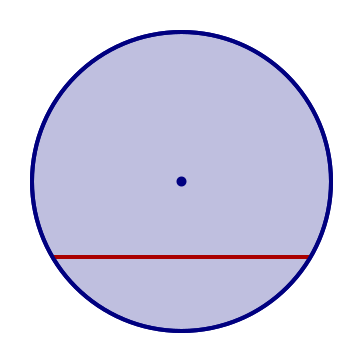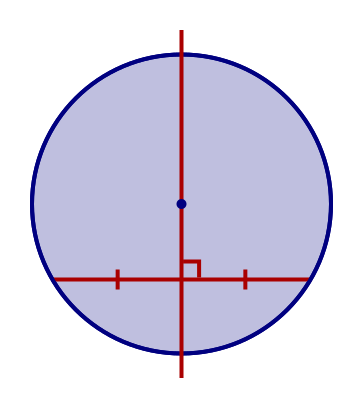# Perpendicular bisector of a chord

By Martin McBride, 2020-08-16
Tags: circle chord perpendicular bisector
Categories: gcse geometryA chord is any line drawn across a circle, from one point on the circumference to another:The perpendicular bisector of a chord is the line that cuts the chord in half, at a right angle:The perpendicular bisector of a chord passes through the centre of the circle.

This theorem is covered in this video on circle theorems:

## Proof

To prove this theorem, we can form a triangle AOB, where O is the centre of the circle, and A and B are the points where the chord meets the circumference:Since OA and OB are both radii of the circle, they are of equal length. This means that the triangle is an isosceles triangle (see the two radii rule):The perpendicular bisector of the side AB (the chord) is an axis of symmetry of the isosceles triangle:We know that the axis of symmetry an isosceles triangle passes through the vertex O, (which is the centre of the circle).

Therefore the perpendicular bisector of the chord passes through the centre of the circle.